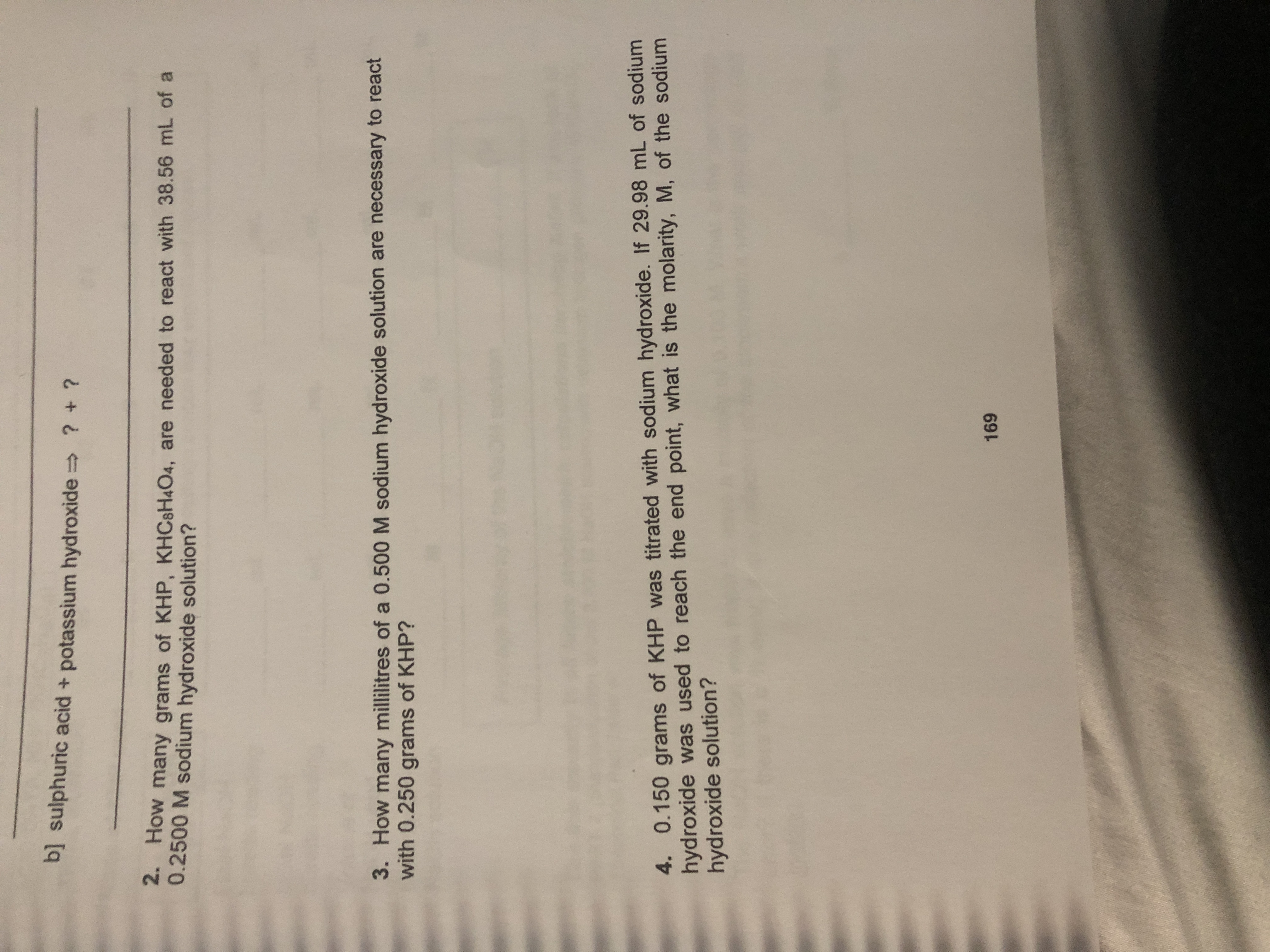# sulphuric acid + potassium hydroxide? +?2. How many grams of KHP, KHCsH4O4, are needed to react with 38.56 mL of a0.2500 M sodium hydroxide solution?3. How many millilitres of a 0.500 M sodium hydroxide solution are necessary to reactwith 0.250 grams of KHP?4. 0.150 grams of KHP was titrated with sodium hydroxide. If 29.98 mL of sodiumhydroxide was used to reach the end point, what is the molarity, M, of the sodiumhydroxide solution?169

Question
6 views

Question 4help_outlineImage Transcriptionclosesulphuric acid + potassium hydroxide ? +? 2. How many grams of KHP, KHCsH4O4, are needed to react with 38.56 mL of a 0.2500 M sodium hydroxide solution? 3. How many millilitres of a 0.500 M sodium hydroxide solution are necessary to react with 0.250 grams of KHP? 4. 0.150 grams of KHP was titrated with sodium hydroxide. If 29.98 mL of sodium hydroxide was used to reach the end point, what is the molarity, M, of the sodium hydroxide solution? 169 fullscreen
check_circle

Step 1

The balanced chemical equation between potassium hydro...

### Want to see the full answer?

See Solution

#### Want to see this answer and more?

Solutions are written by subject experts who are available 24/7. Questions are typically answered within 1 hour.*

See Solution
*Response times may vary by subject and question.
Tagged in

### Halogen Compounds